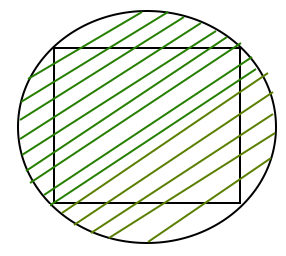Open in App
Not now

# GATE | GATE CS 2018 | Question 5

• Last Updated : 22 Feb, 2018

The area of a square is ‘d’. What is the area of the circle which has the diagonal of the square as its diameter?
(A) πd
(B) πd2
(C) (1/4) πd2
(D) (1/2)πd

Explanation:```One important observation to solve
the question :

Diagonal of Square = Diameter of Circle.

Let side of square be x.

From Pythogorous theorem.
Diagonal = √(2*x*x)

We know area of square = x * x = d

Diameter = Diagonal =  √(2*d)